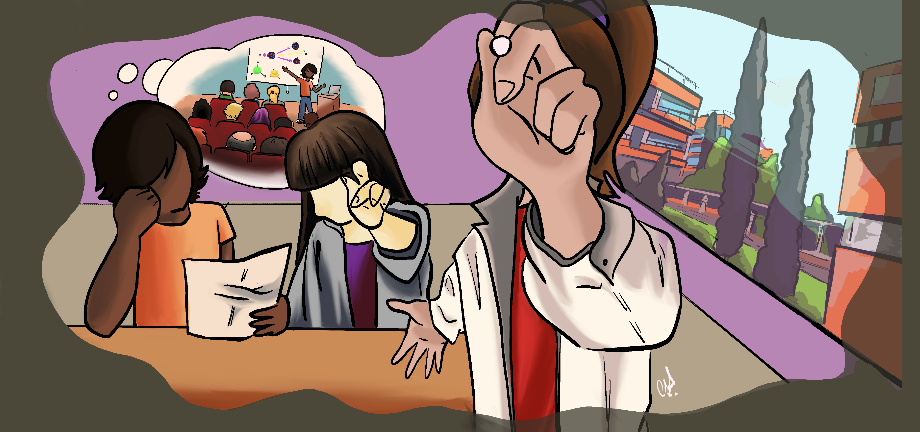#@FlipPhysics

21-25 March 2022
Salon de actos del IATA

## Visible final-state kinematics in $b \to c\tau( \pi\nu_\tau, \rho\nu_\tau, \mu\bar{\nu}_\mu\nu_\tau)$ reactions

Not scheduled
15m
Salon de actos del IATA

#### Salon de actos del IATA

Carrer del Catedràtic Agustín Escardino Benlloch, 7, 46980 Paterna, Valencia
Talk

### Speaker

Neus Penalva Martínez (IFIC (CSIC-UV))

### Description

In the context of lepton flavor universality violation (LFUV) studies, we study different observables related to the $b\to c\tau\bar{\nu}_\tau$ semileptonic decays. These observables are expected to help in distinguishing between different NP scenarios. Since the $\tau$ lepton is very short-lived, we consider three subsequent $\tau$-decay modes, two hadronic $\pi\nu_\tau$ and $\rho\nu_\tau$ and one leptonic $\mu\bar{\nu}_\mu\nu_\tau$, which have been previously studied for $\bar{B}\to D^{(*)}$ decays. This way the differential decay width can be written in terms of visible (experimentally accessible) variables of the massive particle created in the $\tau$ decay.
There are seven different $\tau$ angular and spin asymmetries that are defined in this way and that can be extracted from experiment. In addition to these asymmetries, we study the $d^2\Gamma_d/(d\omega d\cos\theta_d)$, $d\Gamma_d/d\cos\theta_d$ and $d\Gamma_d/dE_d$ distributions.
We present numerical results for the $\Lambda_b\to\Lambda_c\tau\bar{\nu}_\tau$ semileptonic decay, which is being measured with precision at the LHCb.

### Primary author

Neus Penalva Martínez (IFIC (CSIC-UV))

### Co-authors

Dr. Juan Nieves Pamplona (IFIC (CSIC-UV)) Dr. Eliecer Hernández Gajate (U.Salamanca)

### Presentation Materials

###### Your browser is out of date!

Update your browser to view this website correctly. Update my browser now

×